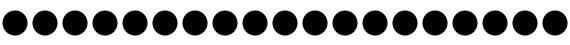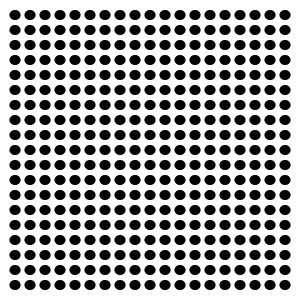## Intro to Loops in Java (Processing)

This tutorial loosley follows what we cover in our Intro to Java classes, and is a great example of what we'll cover in the early days of the class.

### Loops

Loops are a handy way to do something over and over for as few or as many times as you like. Let's say we wanted to draw a row of small circles across our sketch, like so:Yes, you could type the `ellipse` command many times over, but it's just easier to let the computer do the repetitive work for you.  This is where loops come into play.

### `while` loops

The first kind of loop we'll use is a `while` loop:

``````	// declare and initialize in one step:
int num = 1;

// while the statement inside the () is true,
//	execute the code within the {} over and over:
while ( num < 20 )
{
// do something interesting...
ellipse( num * 30, 50, 25, 25 );

// change the value of the variable that controls the while loop:
num = num + 1;
}``````

There are three critical parts to every loop:

• initialization - where you set things up before you start looping.  In the above example, this is the line that initializes things:
``int num = 1;``
Note that we are both declaring and initializing the variable `num`.
• loop test - this is where you test to see if the code inside the loop should run. In the example, this is:
``while ( num < 20 )``
The test happens inside the parenthesis: `num < 20`. This is a boolean statement, that allows the loop to run when it is `true`, and stops the loop when it is `false`. In this case, the test is `true` whenever `num` is less than `20`.
• value change - this is where you increment, or change the value of a variable that will eventually cause the loop to stop:
``num = num + 1;``
Here we're setting the value of `num` to be the current value of `num` plus 1 - or in other words, we're adding one to `num` (note that there's a shortcut for just adding one to a variable: `num++;`). This will eventually cause the loop test to be `false`, and the loop to stop.

The final, and more interesting part of a loop is the rest of the code that you want to run, in the example, it's just a single drawing command:

``		ellipse( num * 30, 50, 25, 25 );``

This draws a circle at `num * 30`, `50` - so, across the canvas, one every 30 pixels.

• Where will the first one be drawn?
• Where will the last one be drawn?
• How many times does the loop run? Why?

### `for` loops

Another kind of loop is a `for` loop. `for` loops have the same three critical elements as `while` loops, just expressed in a different way.  Here's the same example as above, but written as a `for` loop:

``````	for ( int num = 1; num < 20; num++ )
{
ellipse( num * 30, 50, 25, 25 );
}``````

In `for` loops, the initialization, test and change all happen within the parenthesis - separated by semi-colons. You could rewrite the above `for` loop like this, just to separate the three parts (it's silly, but will actually run):

``````	for (
int num = 1;	// initialization
num < 20;		// test
num++			// change
)``````

### Common practices

In general, even though `for` loops and `while` loops are functionally interchangeable (i.e. they do the same thing), you'll use `for` loops for counting things, like "draw 10 squares", and `while` loops for gradually changing something, like "draw squares until I reach the edge of the canvas." A lot of programmers us the variable `i` or `n` as a counter in while loops. You can change multiple variables as you progress through a loop, but you'll usually just test one.  For example - can you figure out what this draws:

``````	float x = 30;
for ( int n = 1; n < 20; n++ )
{
ellipse( x, 50, 25, 25 );
x = x + 30;
}``````

### Try it Yourself

Here are some ideas for you to try on your own:

• Use a loop to draw a bunch of circles that go down the canvas
• Use a loop to draw a diagonal line of circles
• from the top left corner to the bottom right corner
• from the top right corner to the bottom left corner
• use a loop to draw a compound shape (e.g. the three circles at the beginning of this post) in an interesting way
• Change something else besides the position of what you're drawing in the loop

How would you draw something like this?